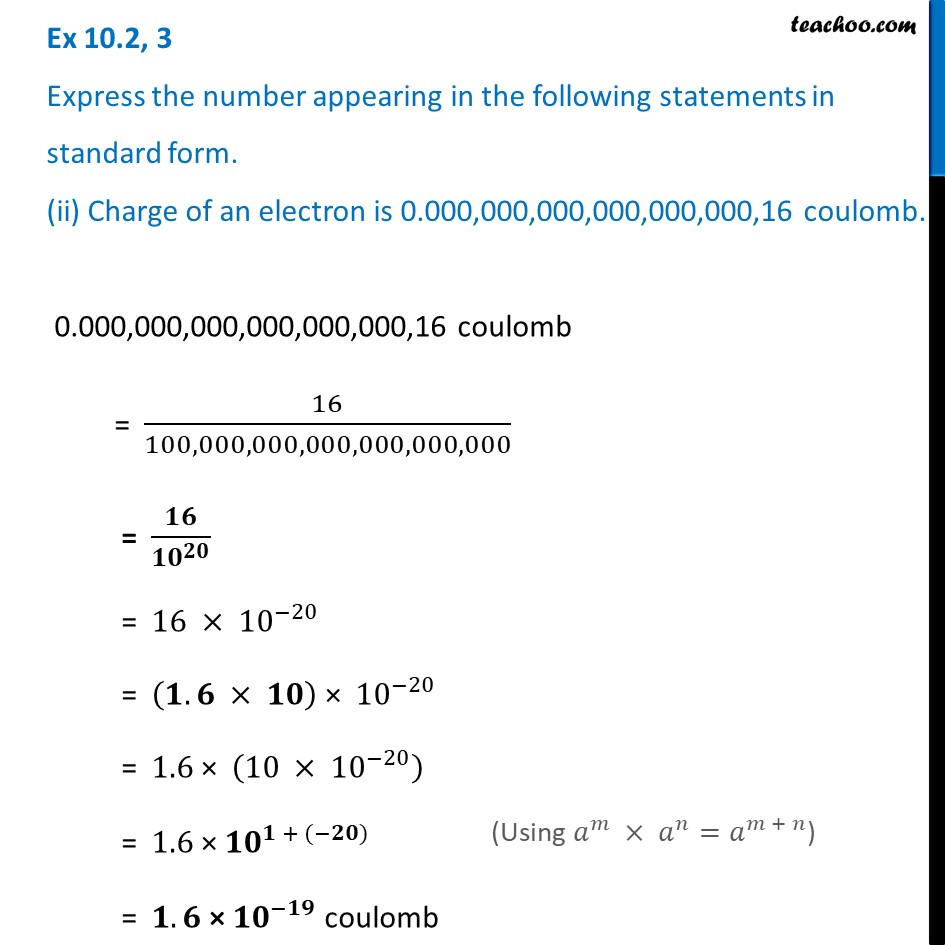Ex 10.2

Chapter 10 Class 8 Exponents and Powers
Serial order wiseLearn in your speed, with individual attention - Teachoo Maths 1-on-1 Class

### Transcript

Ex 10.2, 3 Express the number appearing in the following statements in standard form. (ii) Charge of an electron is 0.000,000,000,000,000,000,16 coulomb. 0.000,000,000,000,000,000,16 coulomb = 16/100,000,000,000,000,000,000 = 𝟏𝟔/〖𝟏𝟎〗^𝟐𝟎 = 16 × 10^(−20) = (𝟏.𝟔 × 𝟏𝟎) × 10^(−20) = 1.6 × (10 × 10^(−20) ) = 1.6 × 〖𝟏𝟎〗^(𝟏 + (−𝟐𝟎)) = 𝟏.𝟔 × 〖𝟏𝟎〗^(−𝟏𝟗) coulomb